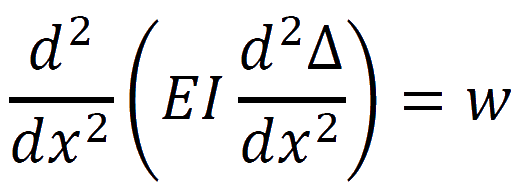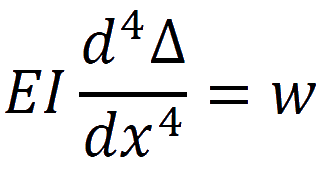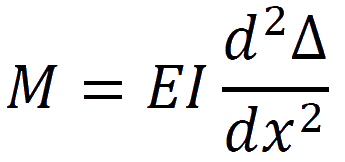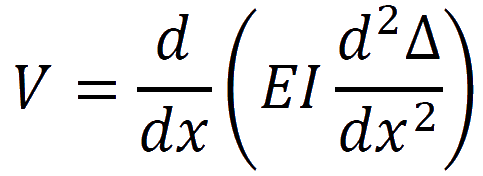# Definition of a beam

A structural element or member subjected to forces and couples along the members longitudinal axis. The member typically spans between one or more supports and its design is generally governed by bending moments.

# Euler-Bernoulli Beam Theory

The Euler-Bernoulli equation describes the relationship between the applied load and the resulting deflection of the beam and is shown mathematically as:Where w is the distributed loading or force per unit length acting in the same direction as y and the deflection of the beam Δ(x) at some position x. E is the modulus of elasticity of the material under consideration and I is the second moment of area calculated with respect to the axis which passes through the centroid of the cross-section and is perpendicular to the applied load. If EI or the flexural rigidity does not vary along the beam then the equation simplifies to:Once the deflection due to a given load has been determined the stresses in the beam can be calculated using the following expressions:

The bending moment in the beam:The shear force in the beam:# Support connections and reactions

There are four different types of connection that are commonly encountered when dealing with beams and each one determines the type of load that the support can resist as well as the overall load bearing capacity of not only the member under consideration but also the system in which the member is a part of.

Roller supports: are free to rotate and translate along the surface upon which the roller rests and as a result are unable to resist lateral forces. Such supports are subjected to a singular reaction force acting perpendicular to and away from the surface.

Pinned Supports: allow the member or beam to rotate (sometimes in only one direction), but not to translate in any direction, that is, they can resist both vertical and horizontal forces but not bending moments.

Fixed Supports: restrain against both rotation and translation and resist both vertical and horizontal forces as well as bending moments.

Simple Supports: are free to rotate and translate along the surface which they rest in all directions but perpendicular to and away from the surface. Simple supports are dissimilar to roller supports in that they cannot resist lateral loads of any magnitude.

# Types of Beams

Simply support beam: freely supported at each end the member is free to rotate at the end bearing points and has no resistance to bending moments. The end supports of the beam are capable of exerting forces on the beam but will rotate as the member deflects under any loads.

Fixed beam: restrained at each end of the member the end points are restricted from rotation and movement in both the vertical and horizontal directions.

Cantilever beam: a member fixed at one end only with the other end free to rotate and move freely in both vertical and horizontal directions.

Overhanging: a simple beam that extends beyond its supports at one or both ends.

Continuous: a beam extending over more than two supports.

# Accuracy of engineer's beam theory

Because of the assumptions, a general rule of thumb is that for most configurations, the equations for flexural stress and transverse shear stress are accurate to within about 3% for beams with a length-to-height ratio greater than 4. The conservative nature of structural design (load factors) in most instances compensate for these inaccuracies. It is also important to understand and to give consideration to the type of material/s which makes up the beam, the way the beam deforms, the geometry of the beam including the cross-sectional area and the internal equilibrium present.

# Assumptions and limitations

• The cross-section of the beam is considered small compared to its length meaning that the beam is long and thin.
• Loads act transverse to the longitudinal axis and pass through the shear centre eliminating any torsion or twist.
• Self-weight of the beam has been ignored and should be taken into account in practice.
• The material of the beam is homogenous and isotropic and has a constant Young's modulus in all directions in both compression and tension.
• The centroidal plane or neutral surface is subjected to zero axial stress and does not undergo any change in length.
• The response to strain is one dimensional stress in the direction of bending.
• Deflections are assumed to be very small compared to the overall length of the beam.
• The cross-section remains planar and perpendicular to the longitudinal axis during bending.
• The beam is initially straight and any deflection of the beam follows a circular arc with the radius of curvature considered to remain large compared to the dimension of the cross section.

# Curved Beams and Arches

Whilst the design of curved beams is identical to that of straight beams when the dimensions of the cross-section are small compared to the radius of curvature the primary difference between curved beams and arches is that the curvature has been increased to a point where axial forces become significant in arches.

# A note about bending moments

In structural engineering the positive moment is drawn on the tension side of the member allowing beams and frames to be dealt with more easily. Because moments are drawn in the same direction as the member would theoretically bend when loaded it is easier to visualise what is happening. StructX has adopted this way of drawing bending moments throughout.

A selection of beam equations along with relevant engineering calculators can be found Here.